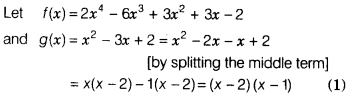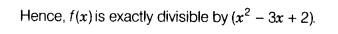# Without actual division, prove that (2x4 -6x3 + 3x2 + 3x-2) is exactly divisible by (x2 -3x + 2)

Without actual division, prove that (2x4 -6x3 + 3x2 + 3x-2) is exactly divisible by (x2 -3x + 2).Now, f(x) will exactly be divisible by g(x), if it is exactly divisible by (x - 2) as well as (x -1).
For this, we must have f(2) = 0 and f(1) = 0∴ f(x) is exactly divisible by (x - 2) as well as (x -1).
So,f(x) is exactly divisible by (x - 2)(x -1).